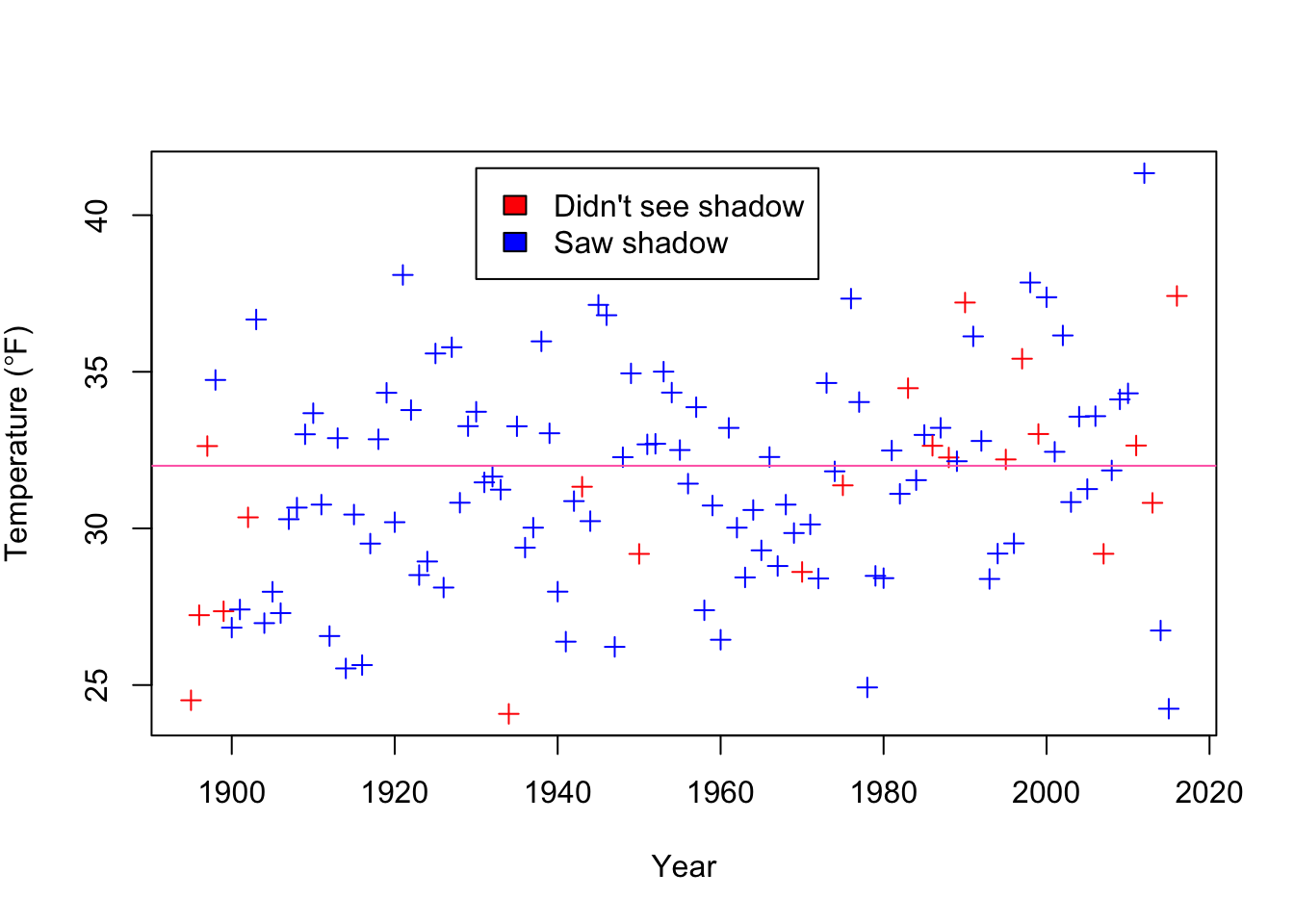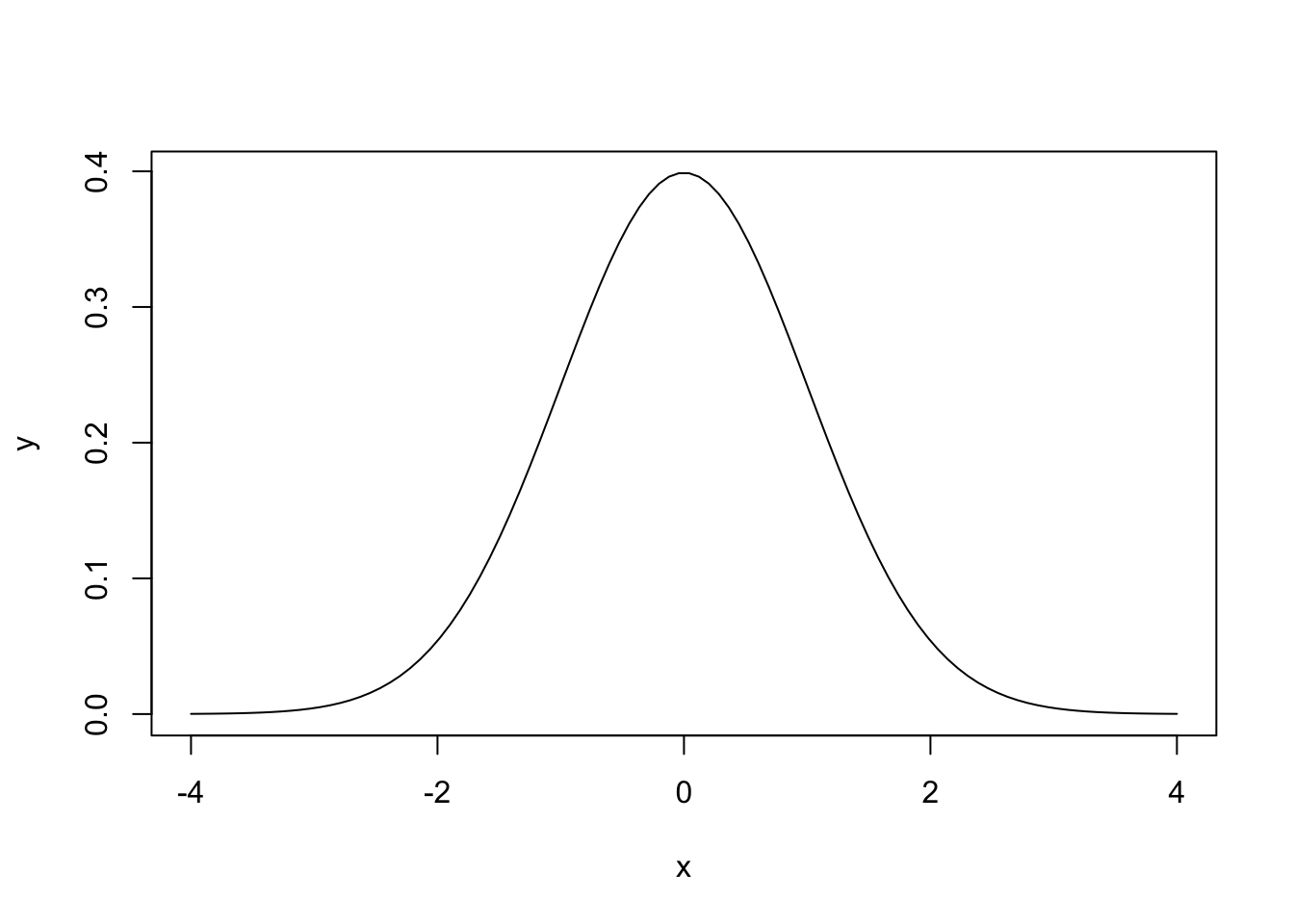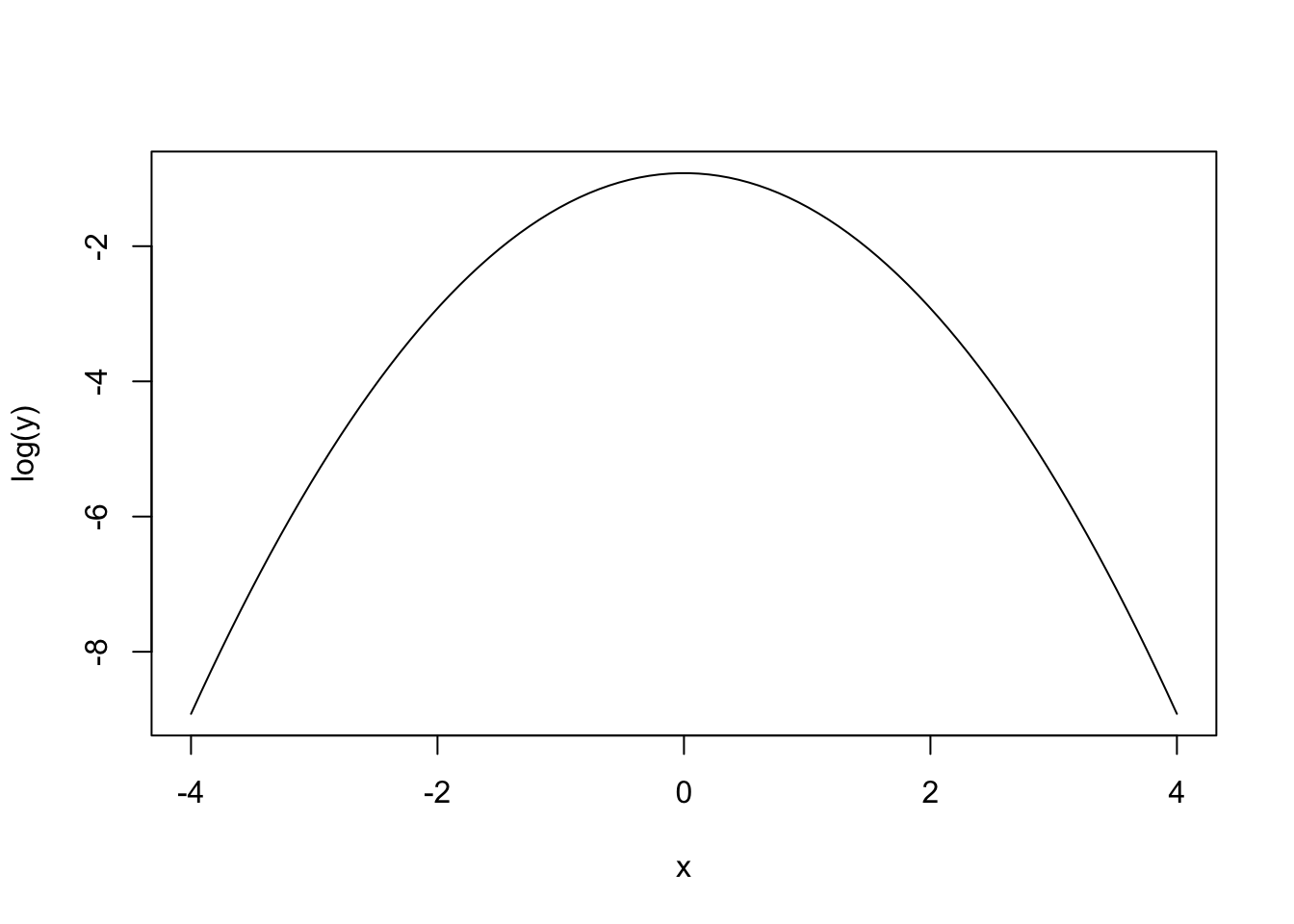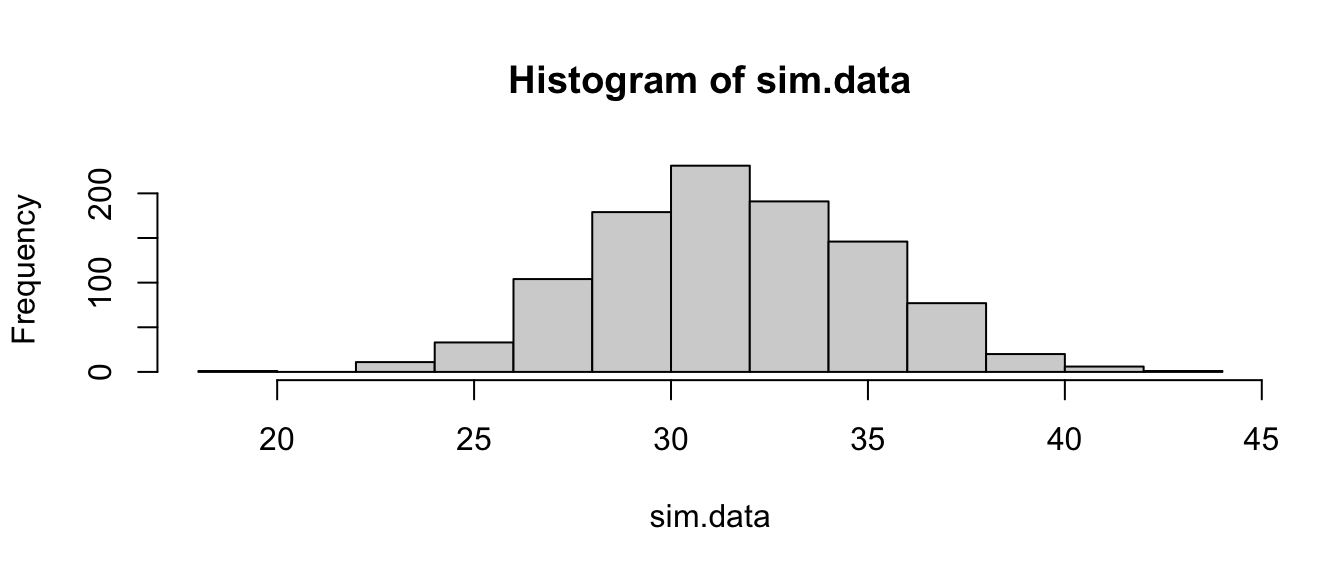## Recap

Begin with the intro video

So far we have

• learned about maximising the likelihood
• estimated confidence intervals and standard errors

These are the basic tools we will use to fit and understand our models

## More than one datum

So far we have only used one data point. But what if we have more? The standard approach starts by making one additional assumption: that each data point is collected independently of the rest. This would mean that each time data is collected, it doesn’t depend on what the last data point was.

If we assume that the data are independent, then from the definition of independence:

$$Pr(X_1 \& X_2) = Pr(X_1) Pr(X_2)$$

So, because the likelihood is the probability of each data point given the parameters, we can calculate the likelihood for the parameters ($$\theta$$) given all of the data ($$X_i$$’s) by multiplying the probabilities:

$Pr(X_1, X_2,...,X_n|\theta) = Pr(X_1|\theta)Pr(X_2|\theta)...Pr(X_n|\theta) = \prod_ {i=1}^{n} Pr(X_i\theta)$

But we usually work on the log-likelihood scale, where we have:

$l(\theta|X_1, X_2,...,X_n) = \sum_{i=1}^{n} \log(Pr(X_i|\theta))$

So, we just add the log-likelihoods together, which is easier than multiplying, and makes a lot of the maths easier.

## Punxsutawney Phil & Groundhog Day

Every 2nd of February, the town of Punxsutawney in the US has a ritual where they go to Gobbler’s Knob, drag a groundhog out of the ground, call it Phil, and ask it if it sees Bill Murray’s its shadow. If Punxsutawney Phil sees his shadow, he’ll decide to retreat, because there will be another 6 weeks of winter.

The data can be found here: https://www.math.ntnu.no/emner/ST2304/2021v/Week04/GroundhogDayta.csv It is a .csv file and can be downloaded AND imported using read.csv() and the full url above.

The question is whether Punxsutawney Phil is actually any good at predicting the weather. We will answer that by looking at whether he sees his shadow, and the temperature for the following 6 weeks.Before getting to the question of whether Phil is any good as a weatherman, we’ll look at the distribution of the temperature. Of the 122 observations, 56 are above freezing (above 32 degrees Fahrenheit as it is the USA). But we want to summarise the data in more detail, partly so we can ask more complicated questions and also so we can develop the theory. We will do this with the normal distribution.

## The Normal Distribution

The normal distribution looks like this:Or, for those who like equations, like this:

$f(x| \mu, \sigma^2) = \frac{1}{\sqrt{2 \pi \sigma^2}} e^{-\frac{(x - \mu)^2}{2\sigma^2} }$ $$x$$ is the thing that follows a normal distribution (e.g. temperature). $$f(x|\mu, \sigma^2)$$ is the density: a higher density means that value of $$x$$ is more likely1. We call $$f(x|\mu, \sigma^2)$$ the probability density function, and use it to calculate the probability that $$x$$ is between two values (e.g. $$Pr(-1.96 < x < 1.96| \mu=0, \sigma^2 = 1) = 0.95$$ ) The parameters are $$\mu$$ and $$\sigma^2$$: the mean and variance (sometikmes we use the standard deviation, $$\sigma$$, rather than $$\sigma^2$$, or even $$1/\sigma^2$$, the precision).

We can split the function up into bits. The first part is

$\frac{1}{\sqrt{2 \pi \sigma^2}}$

which does not depend on $$x$$, so is a constant. It is there because the area under the whole curve has to equal 1. More important is the second part

$e^{-\frac{(x - \mu)^2}{2\sigma^2} }$

If we take the log transformation (so we have the log probability, or later the log likelihood) we see that it is this

$-\frac{(x - \mu)^2}{2\sigma^2}$

So is a quadratic curve, and looks like this:## The Normal Distribution: Exercises

For these exercises you will need a few extra functions. You can find them by using source() to import and run a script found here https://www.math.ntnu.no/emner/ST2304/2021v/Week04/NormalDistFunctions.R

Let’s look at the normal distribution, and some R functions we can use to play with it. In particular:

• rnorm() - this simulates from a normal distribution. Takes arguments: n = number of observations to simulate, mean = mean of the normal distribution you simulate from, sd = standard deviation of the normal distribution you simulation from.
• dnorm() - this calculates the density of a normal distribution for values x. First argument is x, then mean. sd, and log which can = TRUE or FALSE.
• pnorm() - this calculates the cumulative density of a normal distribution for values x. This is the probability that a random draw from the distribution would be less than x. First argument is q = quantiles you want the probability for, then mean and sd.
• qnorm() - this calculates the values of x that would be the qth quantile. First argument is p = probabilities you want x for, then mean and sd.

Simulate 1000 data points from a normal distribution with the same mean and standard deviation as the temperature data, using rnorm() and plot with hist()

R help

You will need to edit this code.

MeanTemp <- mean(YOURDATA$Temperature) sdTemp <- sd(YOURDATA$Temperature)
sim.data <- rnorm(n=5, mean = MeanTemp, sd = sdTemp)
hist(sim.data)

MeanTemp <- mean(GDay$Temperature) sdTemp <- sd(GDay$Temperature)
sim.data <- rnorm(n=1000, mean = MeanTemp, sd = sdTemp)
hist(sim.data)Calculate the density data from the distribution for values using dnorm(), but for more than 4 points and plot it with plot() - why is the density different from the probability (hint: the normal is continuous)

You might also want to use seq() to create the input for dnorm(). This will mean that the numbers are in ascending order rather than mixed like the raw data from the question above.

R help if you need it

# First, create a sequence of numbers to calculate the density on
At.data <- seq(min(YOURDATA$Temperature), # takes the minimum temperature value max(YOURDATA$Temperature), # takes the maximum temperature value
length = 4) # tells R how many to have in the sequence

# create the density data
dens.data <- dnorm(At.data, mean = MeanTemp, sd = sdTemp)

# plot it
plot(x = At.data, y = dens.data, type="l")

The density is different to the probability for the normal distribution because it is a continuous distribution. Therefore, the likelihood of observing any combination of observed data points for a given parameter value is 0 because there are infinite possibilities of observed data. So, instead we take the probability density of observed data points being between two values (often very close together).

# First, create a sequence of numbers to calculate the density on
At.data <- seq(min(GDay$Temperature), # takes the minimum temperature value max(GDay$Temperature), # takes the maximum temperature value
plot(x = At.data, y = dens.data, type="l")